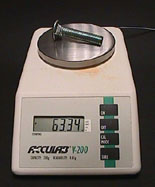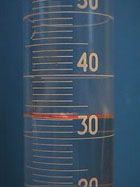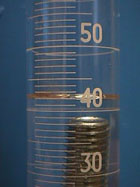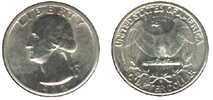Density - Prelab Questions

## Prelab Questions

 1 The data required to calculate the density of a bolt was collected. (Clicking on the picture will show a larger version of it).a. Use this data to determine the density of the bolt. b. Compare the density of the bolt to the density of the nuts in the background section. c. What does this say about the composition of the bolt and the nuts? 2 The density of a liquid was determined by the graphical method described in the background section. Instead of plotting the mass in grams, pounds were used. Does the slope of the line still represent the density of the material? Explain your answer. 3 In 1965, the United States Mint removed all silver (density = 10.5 g/ml) from dimes and quarters. The new coins were made of a copper core (density = 8.9 g/ml) plated with nickel (density = 8.9 g/ml). In appearance, size, and volume, the coins remained identical to those minted before 1964 (see the 1932 quarter below). What happened to the mass of the coins when the change in metals was made?4 The density of a material can be used to calculate the volume of an object if its mass is known. Likewise, the mass can be calculated if the density and volume are known. A pre-1964 quarter, made predominantly of silver (density = 10.5 g/ml), was determined to have a mass of 6.25 g. What is the volume of the quarter?

This completes the prelab. Proceed to the experiment.